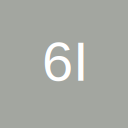# Identifying An Unknown Range

• I'm trying to set a range to a particular cell in Col D but I don't know the row number because this can vary depending on the amount of data entered.

At present my code looks like this

Code
``````Dim MyCount as Integer
Dim r1 as Range
'No of rows in Col D
MyCount = Range("A2:D2", Range("A2:D2").End(xlDown)).Rows.Count
Set r1 = Range(Cells(MyCount, 4))``````

The problem is with my line

Code
``Set r1 = Range(Cells(MyCount, 4))``

Any help much appreciated

• Re: Identifying An Unknown Range

Hi

try

Code
``lr = Activecell.SpecialCells(xlCellTypeLastCell).Row``

HTH

Robert

• Re: Identifying An Unknown Range

To find the next empty cell in a column, I use xlUp, e.g.

Code
``````Dim rNextCl as Range

Set  rNextCl =  Range("d6536").End(xlUp).Offset(1,0)
'or
Set rNextCl = Cells(Rows.Count,4).End(xlUp).Offset(1,0)``````

## Participate now!

Don’t have an account yet? Register yourself now and be a part of our community!